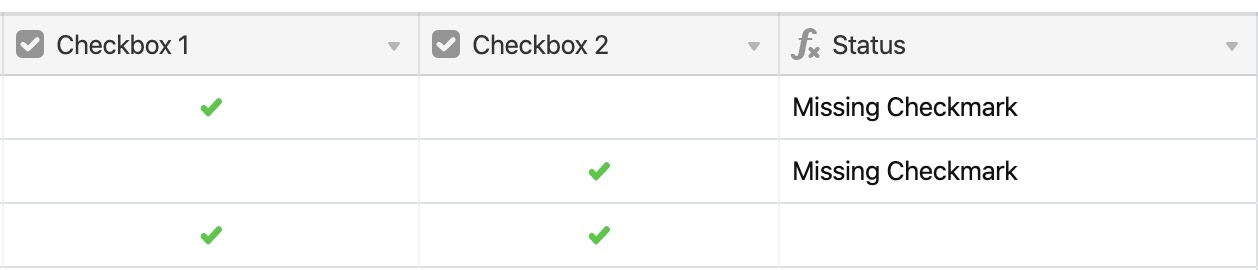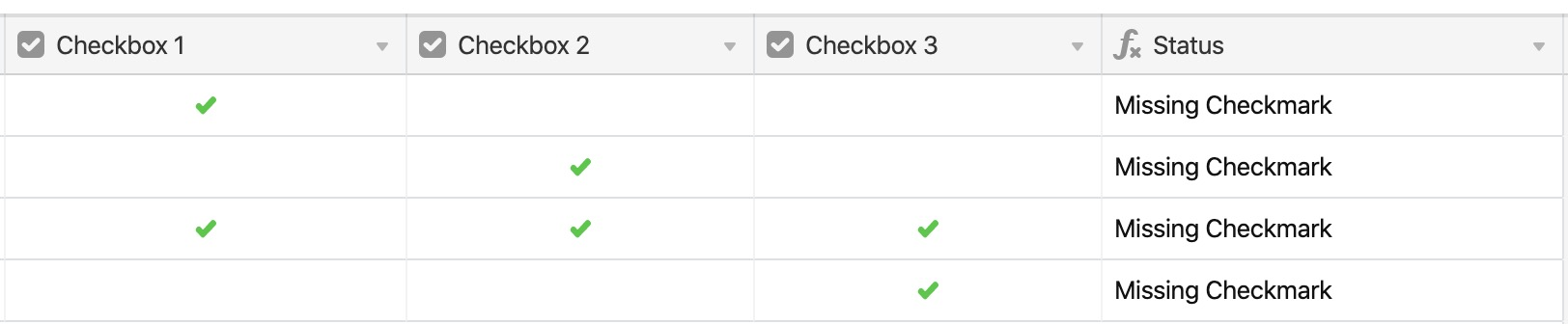• English
• Account
• API
• Integrations
• Enterprise
• Resources
Other logical functions
• 04 Oct 2022
• Dark
Light
• PDF

# Other logical functions

• Dark
Light
• PDF

Article Summary

In this article, we'll walk through how to use the `XOR `and `NOT` functions. This article is part of a guided course which you can view here.

## `XOR()`

`XOR `returns a true value if an odd number of arguments are true. As an example, let's say that you want to make sure that all checkboxes across fields are checked, and output a message if one is missing.

The formula below evaluates for that by checking for an odd number of arguments using `XOR`, and showing a message if that is found.

``````IF(
XOR(
{Checkbox 1},
{Checkbox 2}
),
"Missing Checkmark"
)``````Note that this works because there are an even number of fields - if there were an odd number of fields (say, 3 of them), and you adjusted the formula to check the third field, the results would be incorrect.

``````IF(
XOR(
{Checkbox 1},
{Checkbox 2},
{Checkbox 3}
),
"Missing Checkmark"
)``````Incorrect result due to an odd number of fields In many cases, using other functions like `AND() `can prove more practical than `XOR()`, but it's helpful to be familiar with its functionality and uses.

## `NOT()`

`NOT() `reverses the logical value of its argument. For example, `100 > 75 `would evaluate as true, but if you wrote `NOT(100 > 75)`, it would evaluate as false.